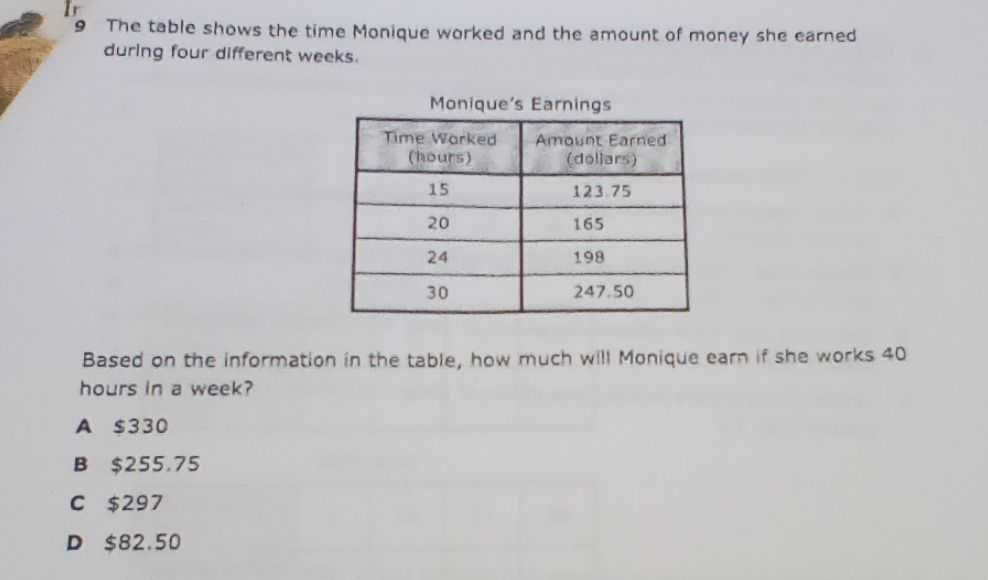### ¿Todavía tienes preguntas de matemáticas?

Pregunte a nuestros tutores expertos
Algebra
Pregunta$$9$$ The table shows the time Monique worked and the amount of money she earned during four different weeks. Based on the information in the table, how much will Monique earn if she works $$40$$ hours in a week?

$$\ 330$$

$$\ 255.75$$

$$\ 297$$

$$\ 82.50$$